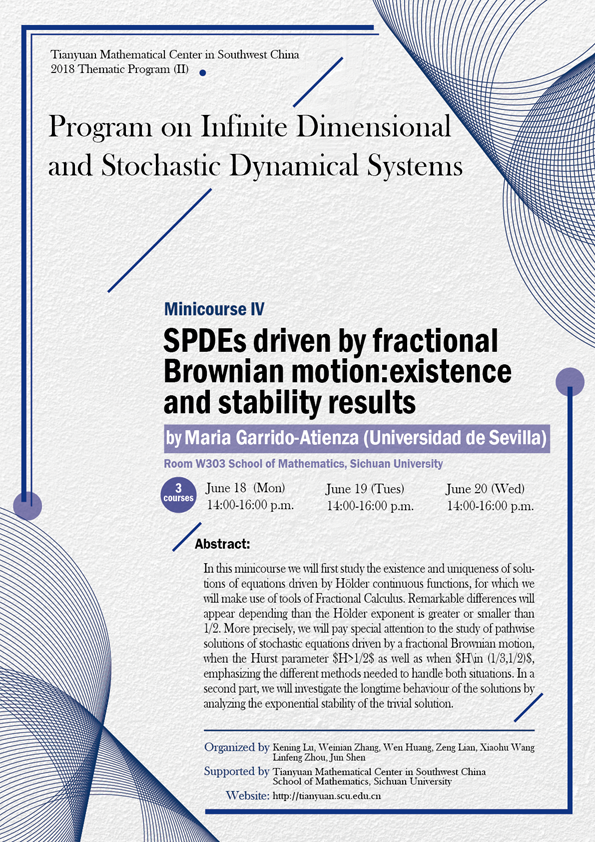2018 Thematic Program (II)

### SPDEs driven by fractional Brownian motion:existence and stability results

#### W303  School of Mathematics, Sichuan University#### ABSTRCT

In this minicourse we will first study the existence and uniqueness of solutions of equations driven by Hölder continuous functions, for which we will make use of tools of Fractional Calculus. Remarkable differences will appear depending than the Hölder exponent is greater or smaller than 1/2. More precisely, we will pay special attention to the study of pathwise solutions of stochastic equations driven by a fractional Brownian motion, when the Hurst parameter $H>1/2$ as well as when $H\in (1/3,1/2)$, emphasizing the different methods needed to handle both situations. In a second part, we will investigate the longtime behaviour of the solutions by analyzing the exponential stability of the trivial solution.

#### ORGANIZERS

Kening Lu (Sichuan University)

Weinian Zhang (Sichuan University)

Wen Huang (Sichuan University)

Zeng Lian (Sichuan University)

Xiaohu Wang (Sichuan University)

Linfeng Zhou (Sichuan University)

Jun Shen (Sichuan University)

#### SUPPORTED BY

Tianyuan Mathematical Center in Southwest China

School of Mathematics, Sichuan University